Courses

Differentiation - Derivatives Notes | Study Engineering Mathematics - GATE

GATE: Differentiation - Derivatives Notes | Study Engineering Mathematics - GATE

The document Differentiation - Derivatives Notes | Study Engineering Mathematics - GATE is a part of the GATE Course Engineering Mathematics.
All you need of GATE at this link: GATE

Derivatives of Implicit Functions

The below example explains the method of derivative of implicit functions.

Example 1: Find dy / dx, if y + sin y = cos x
Solution:
dy / dx + cosy . dy / dx = -sin x
dy / dx (1 + cos y) = -sin x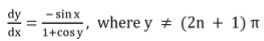n = 0, 1, 2...

Derivatives of Inverse Trigonometric Functions

The below example explains the method of derivative of Inverse Trigonometric functions.

Example 2: Find the value of f’(x) where f(x) = sin-1 x
Solution: Let y = sin-1 x sin y = x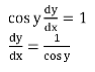We know, sin2y + cos2y = 1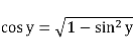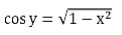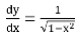Note: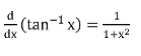Logarithmic Differentiation

The below example explains the method of derivative of Logarithmic functions

Example 3: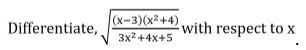Solution:
Let y =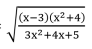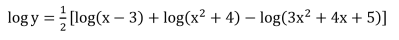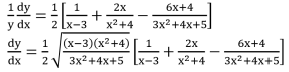Derivatives of Functions in Parametric Forms

The below example explains the method of derivative functions in parametric form.

Example 4: Find, dy / dx, if x = a(θ + sinθ), y = a(1 - cosθ)
Solution:
dx / dθ = a(1 + cosθ)
dy / dθ = a sin θ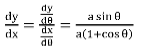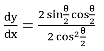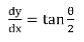Example 5: Find the value of dy / dx if x2 / 3 + y2 / 3 = a2 / 3
Solution:
Let x = a cos3θ and y = a sin3θ which satisfy the above equation
dx / dθ = -3a cos2θ sin θ
dy / dθ = 3a sin2θ cosθ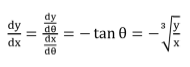Second Order Derivative

So far we have seen only first order derivatives and second order derivative can be obtained by again differentiating first order differential equation with respect to x.
Let y = f (x) then dy / dx = f' (x) ...........(1)
If f’(x) is differentiable, we may differentiate above equation w.r.t x.
i.e. d / dx (dy / dx) is called the second order derivative of w.r.t x and it is denoted by d2y / dx2

Note:

Let the function f(x) be continuous on [a, b] and differentiable on the open interval (a, b), then

1. f(x) is strictly increasing in [a, b] if f’(x) > 0 for each x (a, b)

2. f(x) is strictly decreasing in [a, b] if f’(x) < 0 for each x (a, b)

3. f(x) is constant function in [a, b] if f’(x) = 0 for each x (a, b)

Example 6: Find the intervals in which the function f is given by
f(x) = sin x + cos x : 0 ≤ x ≤ 2π
is strictly increasing or strictly decreasing.
Solution: We have, f(x) = sin x + cos x  f'(x)
= cos x – sin x
Now, f' (x) = 0 gives sin x = cos x which gives that x = π / 4, 5π / 4 in 0 ≤ x ≤ 2π
The point x = π / 4 and x = 5π / 4 divide the interval[0, 2π] into three disjoint intervals
Namely [0, π / 4), (π / 4, 5π / 4) and (5π / 4, 2π]

Note that f’(x) > 0 if x ∈ [0, π / 4) ∪ (5π / 4, 2π] that means f is strictly increasing in this interval

The document Differentiation - Derivatives Notes | Study Engineering Mathematics - GATE is a part of the GATE Course Engineering Mathematics.
All you need of GATE at this link: GATEUse Code STAYHOME200 and get INR 200 additional OFF Use Coupon CodeEngineering Mathematics

54 docs|21 tests

Track your progress, build streaks, highlight & save important lessons and more!

,

,

,

,

,

,

,

,

,

,

,

,

,

,

,

,

,

,

,

,

,

;